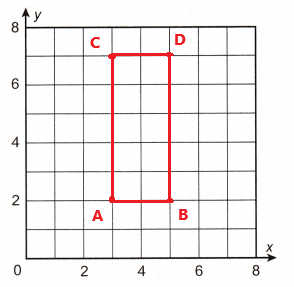All the solutions provided in McGraw Hill Math Grade 5 Answer Key PDF Chapter 11 Posttest are as per the latest syllabus guidelines.

Write each number in standard form.

Question 1.
39 thousandths
The standard form of 39 thousandths is 0.039.

Question 2.
17 and 871 thousandths
The standard form of 17 and 871 thousandths is 17.871.

Question 3.
84 billion, 652 million, 34
The standard form of 84 billion, 652 million, 34 is 84,652,000,034.

Write each number in word form.

Question 4.
7,111,658,314
The word form of the given number 7,111,658,314 is seven billion, one hundred eleven million, six hundred fifty eight thousand, three hundred fourteen.

Question 5.
12,500,500,500
The word form of the given number 12,500,500,500 is twelve billion, five hundred million, five hundred thousand, five hundred.

Write >, <, or = to compare each pair of numbers.

Question 6.
1.1 _______ 0.99
1.1 > 0.99
Explanation:
The decimal number 1.1 is greater than the decimal number 0.99.

Question 7.
0.012 ________ 0.121
0.012 < 0.121
Explanation:
The decimal number 0.012 is less than the decimal number 0.121.

Question 8.
5.687 ________ 5.687
5.687 = 5.687
Explanation:
The decimal number 5.687 is equal to the decimal number 5.687.

Round each number to the place of the underlined digit.

Question 9.
7.123
7.12
Explanation:
Here the number next to the underlined digit is less than five and so the underlined digit will not have any change. So, the rounded number is 7.12.

Question 10.
4.741
5
Explanation:
Here the number next to the underlined digit is greater than five and so 1 is added to the underlined digit. So, the rounded number is 5.

Question 11.
6.148
6.1
Explanation:
Here the number next to the underlined digit is less than five and so the underlined digit will not have any change. So, the rounded number is 6.1.

Question 12.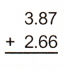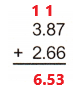Explanation:
Perform addition operation on above two given decimal numbers. Add 3.87 with 2.66 the sum is equal to 6.53.

Question 13.Explanation:
Perform addition operation on above two given numbers. Add 791 with 109 the sum is equal to 900.

Question 14.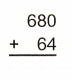Explanation:
Perform addition operation on above two given numbers. Add 680 with 64 the sum is equal to 744.

Question 15.Explanation:
Perform addition operation on above two given decimal numbers. Add 4.72 with 0.16 the sum is equal to 4.88.

Question 16.
61,115 + 17,956 __________
61,115 + 17,956 = 79,071
Explanation:
Perform addition operation on above two given numbers. Add 61,115 with17,956 the sum is equal to 79,071.

Question 17.
8.46 + 0.54 _____________
8.46 + 0.54 = 9.00
Explanation:
Perform addition operation on above two given decimal numbers. Add 8.46 with 0.54 the sum is equal to 9.00.

Question 18.
67.36 + 1.51 _______________
67.36 + 1.51 = 68.87
Explanation:
Perform addition operation on above two given decimal numbers. Add 67.36 with 1.51 the sum is equal to 68.87.

Subtract. Write the difference.

Question 19.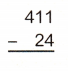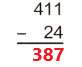Explanation:
Perform subtraction operation on above two given numbers. Subtract 24 from 411 the difference is equal to 387.

Question 20.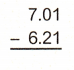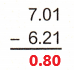Explanation:
Perform subtraction operation on above two given decimal numbers. Subtract 6.21 from 7.01 the difference is equal to 0.80.

Question 21.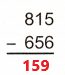Explanation:
Perform subtraction operation on above two given numbers. Subtract 656 from 815 the difference is equal to 159.

Question 22.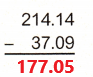Explanation:
Perform subtraction operation on above two given decimal numbers. Subtract 37.09 from 214.14 the difference is equal to 177.05.

Question 23.
77,777 – 3,028 = ___________
77,777 – 3,028 = 74,749
Explanation:
Perform subtraction operation on above two given numbers. Subtract 3,028 from 77,777 the difference is equal to 74,749.

Question 24.
12,499 – 11,645 = ____________
12,499 – 11,645 = 854
Explanation:
Perform subtraction operation on above two given numbers. Subtract 11,645 from 12,499 the difference is equal to 854.

Question 25.
17.66 – 14.76 = _____________
17.66 – 14.76 = 2.90
Explanation:
Perform subtraction operation on above two given decimal numbers. Subtract 14.76 from 17.66 the difference is equal to 2.90.

Question 26.
An alligator’s brain weighs about 0.49 ounces. A peach can weigh about 5.5 ounces. How many ounces heavier is a peach than an alligator’s brain?
An alligator’s brain weighs about 0.49 ounces.
A peach weigh about 5.5 ounces.
Subtract alligator’s brain weight from peach weight.
5.5 ounces – 0.49 ounces = 5.01 ounces
A peach is 5.01 ounces heavier than an alligator’s brain.

Simplify and complete. Tell what property is represented.

Question 27.
15 × 12 = (________ × 12) + (10 × __________) = 60 + __________ = ___________
_____________ Property
15 × 12 = (5 × 12) + (10 × 12) = 60 + 120 = 180
The above property is named as distributive property.
Explanation:
For example take the equation A(B + C). If we want to apply distributive property on the equation A(B + C) we have to multiply A with both B and C and then add.
Distributive property is A (B + C) = A x B + A x C = AB + AC
Here 15 × 12 can be written as (5 + 10)12.
(5 + 10)12 = (5 × 12) + (10 × 12) = 60 + 120 = 180

Question 28.
(8 × 2) × 30 = __________ × (2 × _________) = 8 × ___________ = ___________
______________ Property
(8 × 2) × 30 = 8 × (2 × 30) = 8 × 60 = 480
The above property is named as associative property.

Multiply. Write the product.

Question 29.Explanation:
Perform multiplication operation on above two given numbers. Multiply 256 with 3 the product is equal to 768.

Question 30.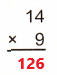Explanation:
Perform multiplication operation on above two given numbers. Multiply 14 with 9 the product is equal to 126.

Question 31.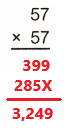Explanation:
Perform multiplication operation on above two given numbers. Multiply 57 with 57 the product is equal to 3,249.

Question 32.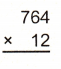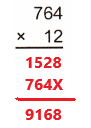Explanation:
Perform multiplication operation on above two given numbers. Multiply 764 with 12 the product is equal to 9,168.

Question 33.
A gym has 2-dozen treadmills. Each treadmill weighs 145 pounds. How many pounds do the treadmills weigh in all?
We know that 1 dozen is equal to 12.
2 x 12 = 24 treadmills
24 x 145 = 3,480 pounds

Question 34.
1,685 × 102 = ______________
1,685 × 102
= 1,685 × 100
= 168,500
Explanation:
Perform multiplication operation on above two given numbers. Multiply 1,685 with 100 the product is equal to 168,500.

Question 35.
59 × 104 = ______________
59 × 104
= 59 x 1,0000
= 590,000
Explanation:
Perform multiplication operation on above two given numbers. Multiply 59 with 1,0000 the product is equal to 590,000.

Question 36.
987 × 101 = ______________
987 × 101
= 987 x 10
= 9,870
Explanation:
Perform multiplication operation on above two given numbers. Multiply 987 with 10 the product is equal to 9,870.

Divide. Write the quotient.

Question 37.
28 ÷ 5 = _____________
28 ÷ 5 = 5.6
Explanation:
Perform division operation on above two given numbers. Here the dividend is 28 and divisor is 5. Divide 28 by 5 the quotient is equal to 5.6.

Question 38.
90 ÷ 2 = _____________
90 ÷ 2 = 45
Explanation:
Perform division operation on above two given numbers. Here the dividend is 90 and divisor is 2. Divide 90 by 2 the quotient is equal to 45.

Question 39.
77 ÷ 8 = ______________
77 ÷ 8 = 9.625
Explanation:
Perform division operation on above two given numbers. Here the dividend is 77 and divisor is 8. Divide 77 by 8 the quotient is equal to 9.625.

Estimate. Then multiply or divide.

Question 40.
Estimate: 3.02 × 4 = ______________
Multiply: 3.02 × 4 = ______________
Estimate:
The estimated product for the expression 3.02 × 4 is 12.
The product for the expression 3.02 × 4 is 12.08.

Question 41.
Estimate: 4.95 × 6 = ______________
Multiply: 4.95 × 6 = ______________
The estimated product for the expression 4.95 × 6 is 27.
The product for the expression 4.95 × 6 is 29.7.

Question 42.
Estimate: 6.14 × 3 = ______________
Multiply: 6.14 × 3 = ______________
The estimated product for the expression 6.14 × 3 is 18.
The product for the expression 6.14 × 3 is 18.42.

Question 43.
15.88 ÷ 102
Estimate: _____________
Quotient: _____________
Here 102 = 100
The estimated quotient for the expression 15.88 ÷ 102 is less than 1.
The quotient for the expression 15.88 ÷ 102 is 0.1588.

Question 44.
872.3 ÷ 104 =
Estimate: _____________
Quotient: _____________
Here 104 = 10,000
The estimated quotient for the expression 872.3 ÷ 104  is greater than 0.08.
The quotient for the expression 872.3 ÷ 104 is 0.08723.

Question 45.
298.4 ÷ 101 =
Estimate: _____________
Quotient: _____________
Here 101 = 10
The estimated quotient for the expression 298.4 ÷ 101  is greater than 29.
The quotient for the expression 298.4 ÷ 101  is 29.84.

Question 46.
1.37 × 102 =
Estimate: _____________
Product: _____________
Here 102 = 100
The estimated product for the expression 1.37 × 102 is 137.
The product for the expression 1.37 × 102 is 137.

Question 47.
7.743 × 104 =
Estimate: _____________
Product: _____________
Here 104 = 10,000
The estimated product for the expression 7.743 × 104 is 77,400.
The product for the expression 7.743 × 104 is 77,430.

Question 48.
15.85 × 103 =
Estimate: _____________
Product: _____________
Here 103 = 1,000
The estimated product for the expression 15.85 × 103  is 15,800.
The product for the expression 15.85 × 103  is 15,850.

Work backward to solve.

Question 49.
Mr. Pedersen is making a pot of marinara sauce. He pours the sauce into 8 jars. Each jar holds 1.5 quarts of sauce. There are 0.75 quarts of sauce left in the pot. How many quarts of sauce were in the pot before Mr. Pedersen began pouring??
He pours the sauce into 8 jars.
One jar holds 1.5 quarts of sauce.
Multiply 1.5 with 8 the product is equal to 12.0 quarts of sauce.
1.5 x 8 = 12.0 quarts
So, 8 jars holds total 12.0 quarts of sauce.
There are 0.75 quarts of sauce left in the pot.
0.75 + 12.0 = 12.75 quarts
12.75 quarts of sauce were in the pot before Mr. Pedersen began pouring.

Simplify and solve. Show your work.

Question 50.
(18 – 8)3 – (12 × 5) × (9 – 4) + 104
Simplify inside the parentheses: _____________
Simplify exponents: _____________
Multiply: _____________
Add and subtract from left to right: _____________
Given equation is (18 – 8)3 – (12 × 5) × (9 – 4) + 104
First simplify inside the parentheses:
(10)3 – (60) × (5) + 104
Second simplifying exponents:
1,000 – 60 × 5 + 10,000
Third performing Multiplication:
1,000 – 300 + 10,000
Fourth performing addition and subtraction from left to right:
700 + 10,000 = 10,700
Final answer is equal to 10,700.

Question 51.
6{[4(1 + 7) + 3] – 15}
Simplify inside the parentheses: _____________
Simplify inside the brackets: _____________
Simplify inside the braces: _____________
6{[4(1 + 7) + 3] – 15} = _____________.
Given 6{[4(1 + 7) + 3] – 15}
First simplifying inside the parentheses:
6{[4(8) + 3] – 15}
Second simplifying inside the brackets:
6{[32 + 3] – 15}
Third simplifying inside the braces:
= 6{ – 15}
= 6{20}
= 120
6{[4(1 + 7) + 3] – 15} = 120

Question 52.
(72 ÷ 8) + (13 – 3)2
Given expression is (72 ÷ 8) + (13 – 3)2
First simplifying inside the parenthesis:
(9) + (10)2
Second simplifying exponents:
(9) + 100
9 + 100 = 109
(72 ÷ 8) + (13 – 3)2 = 109
Question 53.
14 + 42 × (2 × 4) ÷ 2
Given expression is 14 + 42 × (2 × 4) ÷ 2
First simplifying inside the parenthesis:
14 + 42 × (8) ÷ 2
Second simplifying exponents:
14 + 16 × 8 ÷ 2
Third Perform division operation:
14 + 16 x 4
Fourth perform multiplication operation:
14 + 64
14 + 64 = 78

Add or subtract. Give the answers in simplest terms. Change any improper fractions to mixed numbers.

Question 54.
$$\frac{1}{6}$$ + $$\frac{2}{6}$$ _____________
$$\frac{1}{6}$$ + $$\frac{2}{6}$$ = $$\frac{3}{6}$$ or $$\frac{1}{2}$$
Explanation:
Perform addition operation on above two given fraction numbers. Add $$\frac{1}{6}$$ with $$\frac{2}{6}$$ the sum is equal to $$\frac{3}{6}$$. The simplest form of $$\frac{3}{6}$$ is $$\frac{1}{2}$$.

Question 55.
$$\frac{3}{9}$$ + $$\frac{1}{9}$$ _____________
$$\frac{3}{9}$$ + $$\frac{1}{9}$$ = $$\frac{4}{9}$$
Explanation:
Perform addition operation on above two given fraction numbers. Add $$\frac{3}{9}$$ with $$\frac{1}{9}$$ the sum is equal to $$\frac{4}{9}$$.

Question 56.
$$\frac{7}{8}$$ – $$\frac{1}{8}$$ _____________
$$\frac{7}{8}$$ – $$\frac{1}{8}$$ = $$\frac{6}{8}$$ or $$\frac{3}{4}$$
Explanation:
Perform subtraction operation on above two given fraction numbers. Subtract $$\frac{1}{8}$$ from $$\frac{7}{8}$$ the difference is equal to $$\frac{6}{8}$$. The simplest form of $$\frac{6}{8}$$ is $$\frac{3}{4}$$.

Question 57.
$$\frac{4}{9}$$ + $$\frac{5}{6}$$ _____________
$$\frac{4}{9}$$ + $$\frac{5}{6}$$ = $$\frac{23}{18}$$
Explanation:
Perform addition operation on above two given fraction numbers. Add $$\frac{4}{9}$$ with $$\frac{5}{6}$$ the sum is equal to $$\frac{23}{18}$$. The improper fraction $$\frac{23}{18}$$ is converted to mixed number 1$$\frac{5}{18}$$

Question 58.
$$\frac{1}{4}$$ – $$\frac{1}{8}$$ _____________
$$\frac{1}{4}$$ – $$\frac{1}{8}$$ = $$\frac{1}{8}$$
Explanation:
Perform subtraction operation on above two given fraction numbers. Subtract $$\frac{1}{8}$$ from $$\frac{1}{4}$$ the difference is equal to $$\frac{1}{8}$$.

Question 59.
$$\frac{5}{7}$$ – $$\frac{2}{9}$$ _____________
$$\frac{5}{7}$$ – $$\frac{2}{9}$$ = $$\frac{31}{63}$$
Explanation:
Perform subtraction operation on above two given fraction numbers. Subtract $$\frac{2}{9}$$ from $$\frac{5}{7}$$ the difference is equal to $$\frac{31}{63}$$.

Question 60.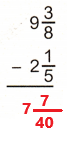Explanation:
The mixed fraction 9$$\frac{3}{8}$$ is converted into fraction form as $$\frac{75}{8}$$.
The mixed fraction 2$$\frac{1}{5}$$ is converted into fraction form as $$\frac{11}{5}$$.
Subtract the fraction $$\frac{11}{5}$$ from $$\frac{75}{8}$$ the difference is equal to $$\frac{287}{40}$$.
The fraction $$\frac{287}{40}$$ is converted into mixed fraction form 7$$\frac{7}{40}$$.

Question 61.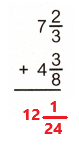Explanation:
The mixed fraction 7$$\frac{2}{3}$$ is converted into fraction form as $$\frac{23}{3}$$.
The mixed fraction 4$$\frac{3}{8}$$ is converted into fraction form as $$\frac{35}{8}$$.
Add the fraction $$\frac{23}{3}$$ with $$\frac{35}{8}$$ the sum is equal to $$\frac{289}{24}$$.
The fraction $$\frac{289}{24}$$ is converted into mixed fraction form 12$$\frac{1}{24}$$.

Question 62.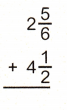Explanation:
The mixed fraction 2$$\frac{5}{6}$$ is converted into fraction form as $$\frac{17}{6}$$.
The mixed fraction 4$$\frac{1}{2}$$ is converted into fraction form as $$\frac{9}{2}$$.
Add the fraction $$\frac{17}{6}$$ with $$\frac{9}{2}$$ the sum is equal to $$\frac{44}{6}$$ or $$\frac{22}{3}$$.
The fraction $$\frac{22}{3}$$ is converted into mixed fraction form 7$$\frac{1}{3}$$.

Question 63.Explanation:
The mixed fraction 5$$\frac{2}{7}$$ is converted into fraction form as $$\frac{37}{7}$$.
The mixed fraction 3$$\frac{3}{4}$$ is converted into fraction form as $$\frac{15}{4}$$.
Subtract the fraction $$\frac{15}{4}$$ from $$\frac{37}{7}$$ the difference is equal to $$\frac{43}{28}$$.
The fraction $$\frac{43}{28}$$ is converted into mixed fraction form 1$$\frac{15}{28}$$.

Find the equivalent fraction.

Question 64.
equivalent to $$\frac{2}{3}$$, denominator 9 _____________
Multiply numerator and denominator with 3.
The fraction equivalent to $$\frac{2}{3}$$ with denominator 9  is $$\frac{6}{9}$$.

Question 65.
equivalent to $$\frac{1}{2}$$, denominator 20 _____________
Multiply numerator and denominator with 10.
The fraction equivalent to $$\frac{1}{2}$$ with denominator 20  is $$\frac{10}{20}$$.

Question 66.
equivalent to $$\frac{1}{4}$$, denominator 28 _____________
Multiply numerator and denominator with 7.
The fraction equivalent to $$\frac{1}{4}$$ with denominator 28  is $$\frac{7}{28}$$.

Question 67.
equivalent to $$\frac{7}{8}$$, denominator 16 _____________
Multiply numerator and denominator with 2.
The fraction equivalent to $$\frac{7}{8}$$ with denominator 16  is $$\frac{14}{16}$$.

Multiply.

Question 68.
24 × $$\frac{1}{6}$$ ______________
24 × $$\frac{1}{6}$$ = 4 x 1 = 4
Explanation:
Perform multiplication operation on above numbers. Multiply 24 with $$\frac{1}{6}$$ the product is equal to 4.

Question 69.
$$\frac{5}{8}$$ × 21 _______________
$$\frac{5}{8}$$ × 21 = $$\frac{105}{8}$$ or 13$$\frac{1}{8}$$
Explanation:
Perform multiplication operation on above numbers. Multiply $$\frac{1}{6}$$ with 21 the product is equal to 13$$\frac{1}{8}$$.

Question 70.
7 × $$\frac{1}{7}$$ _______________
7 × $$\frac{1}{7}$$ = $$\frac{7}{7}$$ = 1
Explanation:
Perform multiplication operation on above numbers. Multiply 7 with $$\frac{1}{7}$$ the product is equal to 1.

Solve.

Question 71.
A florist is making an arrangement of 45 roses. The customer has asked that $$\frac{2}{3}$$ of the roses be pink. How many pink roses does the florist need?
A florist is making an arrangement of 45 roses.
The customer asked that $$\frac{2}{3}$$ of the roses should be pink.
Multiply 45 roses with $$\frac{2}{3}$$ the product is equal to 30 pink roses.
45 x $$\frac{2}{3}$$ = 15 x 2 = 30 pink roses
The florist need 30 pink roses.

Question 72.
$$\frac{3}{4}$$ of Diego’s grocery cart is filled with fresh produce, $$\frac{6}{7}$$ of the produce is leafy greens. What fraction of the produce is leafy greens?

Question 73.
Chase’s journal has 200 pages. So far, he has written 32 pages of journal entries. If he writes $$\frac{1}{2}$$ a page every day, how many days will it take him to fill the remaining pages?
Chase’s journal has 200 pages.
So far, he wrote 32 pages of journal entries.
He writes $$\frac{1}{2}$$ a page every day.
Subtract 32 pages from 200 pages the difference is equal to 168 pages.
200 – 32 = 168 pages
$$\frac{1}{2}$$ page = 1 day
168 pages = ? days
(168 x 1)/$$\frac{1}{2}$$ = 168 x 2 = 336 days
He will take 336 days to fill the remaining pages.

Multiply to find the area of each rectangle.

Question 74.
9 ft long × $$\frac{3}{8}$$ ft wide
area = ________________
To calculate area of a rectangle we need to perform multiplication operation.
Area of a rectangle = 9 ft × $$\frac{3}{8}$$ ft
= $$\frac{27}{8}$$ square feet
= 3$$\frac{3}{8}$$ square feet
Area of a rectangle is equal to 3$$\frac{3}{8}$$ square feet.

Question 75.
5 m long × $$\frac{1}{3}$$ m wide
area = _______________
To calculate area of a rectangle we need to perform multiplication operation.
Area of a rectangle = 5 m× $$\frac{1}{3}$$ m
= $$\frac{5}{3}$$ square meter
= 1$$\frac{2}{3}$$ square meter
Area of a rectangle is equal to 1$$\frac{2}{3}$$ square meter.

Find each quotient. Draw models to help. Use multiplication to check your answers.

Question 76.
$$\frac{1}{6}$$ ÷ 9 = _______________
$$\frac{1}{6}$$ ÷ 9 = $$\frac{1}{54}$$
To check the answer we need to perform multiplication. Multiply $$\frac{1}{54}$$ with 9 the product is equal to $$\frac{1}{6}$$. So, the answer is correct.

Question 77.
$$\frac{1}{2}$$ ÷ 7 = _______________
$$\frac{1}{2}$$ ÷ 7 = $$\frac{1}{14}$$
To check the answer we need to perform multiplication. Multiply $$\frac{1}{14}$$ with 7 the product is equal to $$\frac{1}{2}$$. So, the answer is correct.

Question 78.
9 ÷ $$\frac{1}{8}$$ = _______________
9 ÷ $$\frac{1}{8}$$ = 72
To check the answer we need to perform multiplication. Multiply 72 with $$\frac{1}{8}$$ the product is equal to 9. So, the answer is correct.

Solve.

Question 79.
The reptile exhibit at a zoo has a python that is 5 meters long. There is also a coral snake that is 0.6 meters long. How much longer in centimeters is the python than the coral snake?
A python is 5 meters long.
A coral snake is 0.6 meters long.
We know that 1 meter is equal to 100 cm.
So, 5 meters is equal to 500 cm.
5 x 100 = 500 cm
So, 0.6 meters is equal to 60 cm.
0.6 x 100 = 60 cm
Subtract 60 cm from 500 cm the difference is equal to 440 cm.
500 – 60 = 440 cm
The python snake is 440 cm longer than the coral snake.

Question 80.
People must be at least 54 inches tall to ride a roller coaster at an amusement park. Gabrielle is 4 feet 3 inches tall. Is she tall enough to ride the coaster? How do you know?
To ride a roller coaster at an amusement park people must be at least 54 inches.
Gabrielle is 4 feet 3 inches tall.
We know that 1 feet is equal to 12 inches.
4 feet = 4 x 12 = 48 inches
48 inches + 3 inches = 51 inches
Gabrielle is 51 inches tall.
No, she is 3 inches shorter than the height she need to ride the coaster.

Question 81.
Yolanda is 1 year, 5 days old. Her brother Benjamin is 4 years, 1 week old. How many days older is Benjamin than Yolanda?
We know that 1 year is equal to 365 days.
Yolanda is 1 year, 5 days old.
1 year, 5 days = 365 + 5 = 370 days
Yolanda is 370 days old.
Her brother Benjamin is 4 years, 1 week old.
4 years = 4 x 365 = 1,460 days
We know that 1 week is equal to 7 days.
4 years, 1 week = 1,460 + 7 = 1,467 days
Her brother Benjamin is 1,467 days old.
1467 – 370 = 1097 days
Benjamin is 1097 days older than Yolanda.

Question 82.
What temperature does the thermometer show?The average temperature in Paris in July is 75?F Is the temperature shown by the thermometer higher or lower than average? How many degrees higher or lower?
The thermometer shows the temperature 87℉.
The average temperature in Paris in July is 75℉.
The temperature shown by the thermometer is higher than average.
87℉ – 75℉ = 12℉
12℉ higher.

Use the diagram for Exercises 83 to 85.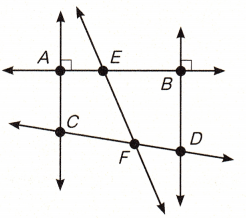Question 83.
Identify 3 points. ________________________
The three points are Point A, Point C, Point F.

Question 84.
Identify 2 intersecting lines that are not perpendicular. __________________________
The two intersecting lines that are not perpendicular are AB and EF.

Question 85.
Identify 3 line segments. _________________
A line segment is part of a line and it has two end points.
One line segment is AE.
Second line segment is CF.
Third line segment is EB.

Classify each angle as straight, right, obtuse, or acute. If you have a protractor, measure each angle. You may need to extend the angle to measure it. Write each measure.

Question 86.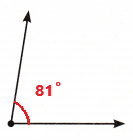Explanation:
In the above image we can observe the angle is 81 degrees. The angle which measures less than 90 degrees is called as acute angle. So, the above angle is acute angle.

Question 87.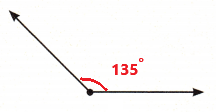In the above image we can observe the angle is 135 degrees. The angle which measures greater than 90 degrees and less than 180 degrees is called as obtuse angle. So, the above angle is obtuse angle.

Place a check mark below the figures that are not polygons.

Question 88.Explanation:
Oval shape and incomplete hexagon are not polygons. So, placed a check mark below the respected figures.

Question 89.
Anthony says that trapezoids are parallelograms because trapezoids have a pair of parallel sides. Do you agree? Why or why not?
No, I don’t agree with Anthony statement because a parallelogram has two pairs of parallel sides and trapezoid have one pair of parallel sides.

Question 90.
Find the volume of the object.Explanation:
First we have to separate the given object into two solids then we have to find the volume of each solid.
Volume of one solid:
Length (l) = 12 cm
Width (w) = 6 cm
height (h) = 8 cm
Volume (v) = (l x w) x h
v = (12 cm x 6 cm) x 8 cm
v = 576 cubic centimeters
Volume of another solid:
Length (l) = 6 cm
Width (w) = 6 cm
height (h) = 8 cm
Volume (v) = (l x w) x h
v = (6 cm x 6 cm) x 8 cm
v =288 cubic centimeters
Now we have to add the two volumes.
576 cubic cm + 288 cubic cm = 864 cubic cm
The volume of the object is 864 cubic cm.

Question 91.
The list of data shows the mass in kilograms of 6 flamingos at a zoo. Draw a line plot to show this data set. Then draw a circle around the outlier.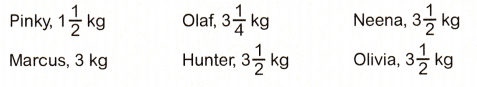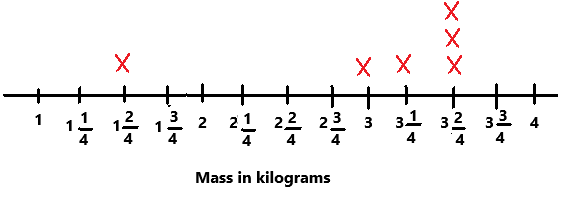Explanation:
The above line plot is numbered from 1 to 4 with an interval of 1/4.
The given data is marked on the line plot with X on top of it.

Plot each set of points. Draw lines between the points. Identify the shape.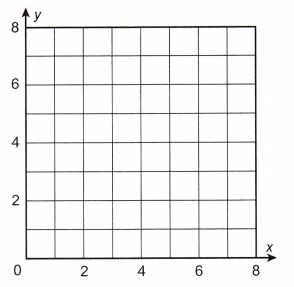Question 92.
A (3, 2)
B (5, 2)
C (3, 7)
D (5, 7)
Identify the shape: __________________